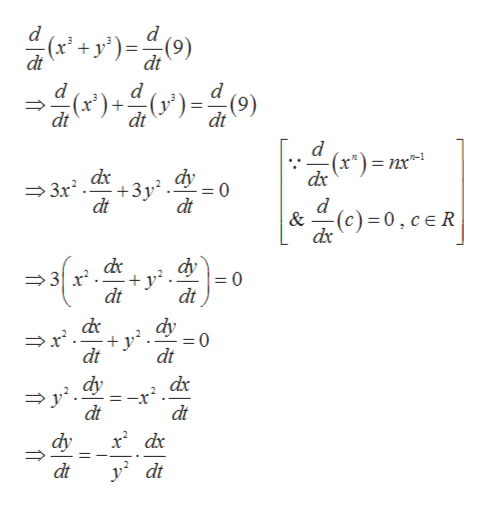# If x3 + y3 = 9 and dx/dt = -3, then what is dy/dt when x = 1 and y = 2?

Question

If x3 + y3 = 9 and dx/dt = -3, then what is dy/dt when x = 1 and y = 2?

check_circleExpert Solution
Step 1

Given,

Step 2

Differentiating with res...help_outlineImage Transcriptionclose블다가들이 ))) -(9) dt d d (6) d (x)+ (1 dt dt (x")=nx dx dy +3y2 dt 3r2 d dt & (c) 0,ceR dx dy +y2 =0 dt dt dy dt dt dy dt dt dy dt fullscreen

### Want to see the full answer?

See Solution

#### Want to see this answer and more?

Solutions are written by subject experts who are available 24/7. Questions are typically answered within 1 hour*

See Solution
*Response times may vary by subject and question
Tagged in

### Derivative